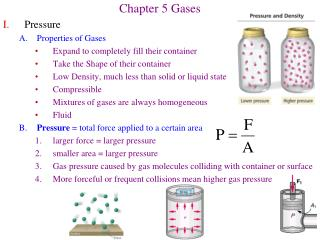Download PresentationChapter 5 Gases

# Chapter 5 Gases

Download Presentation## Chapter 5 Gases

- - - - - - - - - - - - - - - - - - - - - - - - - - - E N D - - - - - - - - - - - - - - - - - - - - - - - - - - -
##### Presentation Transcript

1. Chapter 5 Gases • Pressure • A. Properties of Gases • Expand to completely fill their container • Take the Shape of their container • Low Density, much less than solid or liquid state • Compressible • Mixtures of gases are always homogeneous • Fluid • B. Pressure = total force applied to a certain area • 1. larger force = larger pressure • 2. smaller area = larger pressure • 3. Gas pressure caused by gas molecules colliding with container or surface • 4. More forceful or frequent collisions mean higher gas pressure

2. Measuring Pressure • Atmospheric Pressure is constantly present • Decreases with altitude because of less air • Varies with weather conditions • Measuring pressure using a barometer • Column of mercury supported by air pressure • Longer mercury column = higher pressure • Force of the air on the surface of the mercury is balanced by the pull of gravity on the column of mercury • Units of Gas Pressure • atmosphere (atm) = 29.92 in Hg = 760 mm Hg • Torr = mm Hg 1 atm = 760 torr • Pascal (Pa) = 1 N/m2 1 atm = 101,325 Pa • Example: Convert 49 torr to atm and Pa

3. 5. Measuring the Pressure of a Trapped Gas Sample • Use an manometer to compare to atmospheric pressure • Open-end manometer • if gas end lower than open end, Pgas = Pair + h • if gas end higher than open end, Pgas = Pair – h

4. Simple Gas Laws • A. Boyle’s Law = Pressure is inversely proportional to Volume (constant T, n) • PV = k or P1V1 = P2V2 (k = Boyle’s Law Constant) • As Pressure on a gas increases, the Volume decreases • Example: What is new V of 1.53L of SO2 at 5600Pa when changed to 15,000Pa? V = k(1/P)

5. B. Charles’ Law = V is directly proportional to T (constant P, n) • V = bT • Another way to write Charles Law • As the temperature decreases, the volume of a gas decreases as well • Absolute Zero • Theoretical temperature at which a gas would have zero V and P • 0 K = -273.2 °C = -459 °F (K = oC + 273) • All gas law problems use Kelvin temperature scale! • Example: V = ? If 2.58L of gas at 15 oC is heated to 38 oC at same P?

6. Avogadro’s Law = V directly proportional to moles of gas (Constant T, P) • V = an • Another way to write Avagodro’s Law: • More gas molecules = larger volume • Count number of gas molecules by moles = n • One mole of any gas occupies 22.414 L (at 1 atm, 0 oC) = molar volume • Equal volumes of gases contain equal numbers of molecules • Example: V of O3 = ? If we convert 11.2L (0.50 mol) O2 to O3? • 3O2(g) -----> 2O3(g)

7. Ideal Gas Law • By combining the constants from the 3 gas laws we can write a general equation • Each simple gas law holds something constant • To consider changes in P, V, T, n at the same time, we combine constants • PV = nRT is called the Ideal Gas Law • R is called the gas constant • The value of R depends on the units of P and V • Generally use R = 0.08206Latm/Kmol when P in atm and V in L • Only “Ideal gases” obey this law exactly • Most gases obey when P is low (< 1 atm) and T is high (> 0°C) • An ideal gas is only a hypothetical substance • Constant conditions drop out of the equation to give simpler laws • If you hold n constant

8. Ideal Gas Law Problems • Example: How many moles of H2 gas if: 8.56L, 0 oC, 1.5atm? • Example: P = ? if 7.0 ml gas at 1.68 atm is compressed to 2.7 ml?

9. Example: V = ? If 345 torr, -15 oC, 3.48L changes to 36 oC, 468torr? • 4. Example: DV = ? If 0.35mol, 13oC, 568torr changes to 56oC, 897torr

10. Gas Stoichiometry • Molar volume = volume one mole of any gas occupies • Standard Temperature and Pressure (STP) are 0 oC and 1atm • Real gases differ only slightly from the ideal molar volume • Example: n = ? For 1.75L of N2 at STP? • Example: V(CO2) = ? If 152g CaCO3 decomposes to CaO+CO2? • CaCO3(s) -------> CaO(s) + CO2(g) • Assume ideal gas behavior for CO2

11. Example: • 2.80 L of CH4 at 25 oC and 1.65atm • 35.0L of O2 at 31 oC and 1.25 atm • What is the volume of CO2 formed at 2.50 atm and 125 oC? • CH4(g) + 2O2(g) -------> CO2(g) + 2H2O(g) • Find Limiting reagent • Calculate the amount of CO2 product based on CH4 as limiting reagent • Use the ideal gas law to find the volume of this amount of CO2

12. 7. Calculating Molar Mass of a Gas from density Example: • a. • Example: d = 1.95g/L at 1.50atm and 27 oC. Molar Mass = ? • Assume we have 1.0L of this gas • Calculate Molar Mass from its definition